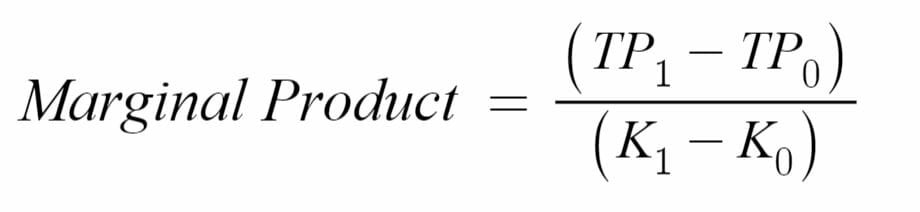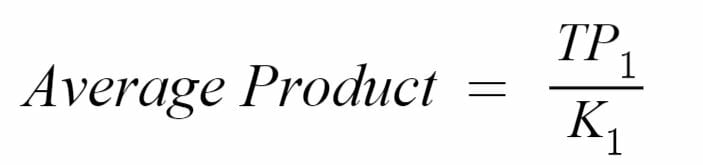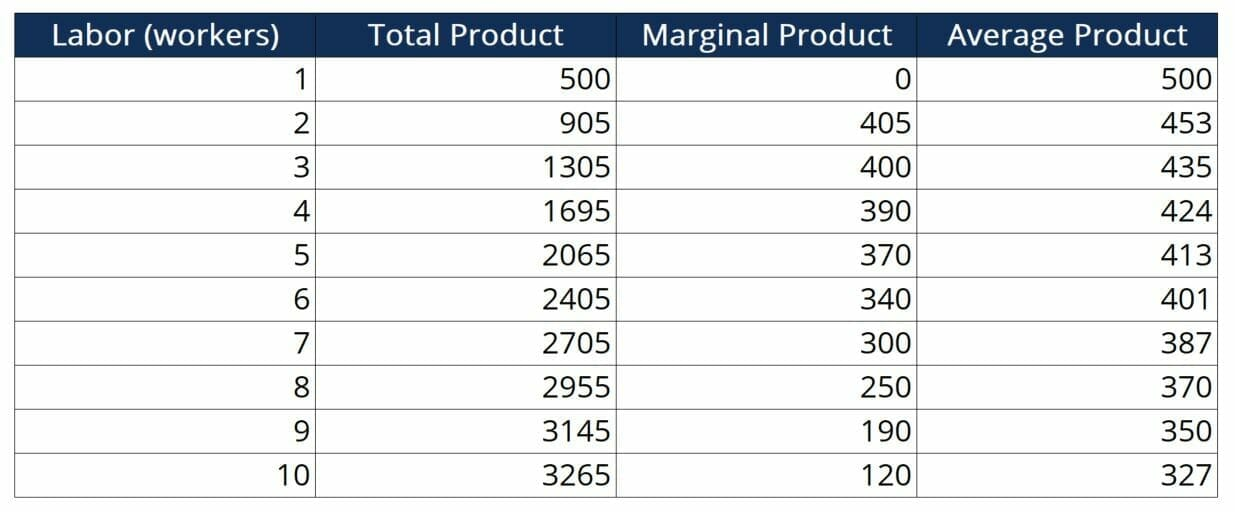# Economics of Production

Understanding total, marginal and average product

## What is Economics of Production?

Production refers to the number of units a firm outputs over a given period of time. From a microeconomics standpoint, a firm that operates efficiently should attain sound knowledge of its total product, marginal product, and average product. In practice, firms can utilize the figures as metrics to make better operational decisions.### Short-Run and Long-Run

Short run production refers to the total output that a firm can achieve in a small time period. The length of the time period depends on how quickly a firm can make changes to fixed manufacturing factors such as machinery, factory size, management, or number of plants. In the short run, firms can manipulate variable manufacturing factors such as labor, overtime, or raw materials to adjust the level of production.

Long run production refers to the output that a firm can achieve after making changes to their machinery, factories, factory size, capital structure, etc. These factors require more time to manipulate than variable manufacturing inputs and, thus, are considered to be “fixed” in the short run.

Consider an oil refinery looking to increase its gasoline output. In the short run, the refinery could hire more workers, incentivize overtime, or purchase larger amounts of crude oil to supplement manufacturing and increase capacity utilization. However, if such measures are insufficient to reach the desired output level, the refinery would need to change its fixed manufacturing factors. For instance, it can purchase additional machinery, expand the current factory space, or invest in new technology.

### Indicators of Production

There are three main metrics that a firm can use to track their output:

##### Total Product (TP)

Total product is the total number (quantity) of units of output that a firm produces per unit time.

##### Marginal Product (MP)

Marginal product refers to the number of additional units that can be produced when the firm has access to one additional unit of a certain factor of production. Thus, marginal product is given in terms of the factor of production that it is describing. For example, if we were measuring how much output would increase by if the firm used one additional unit of labor (one additional worker), we would be measuring the Marginal Product of Labor (MPL). Almost all factors of production face diminishing marginal product, meaning that each additional unit of the factor will lead to less additional production. The equation to calculate MP is as follows:Where:

TP1 – Total Product at time 1

TP0 – Initial total product

K0 – Initial number of units of the factor of production

K1 – Number of units of the factor of production at time 1

##### Average Product (AP)

Average Product is the Total Product divided by the number of units of a certain factor of production. Like the marginal product, average product is expressed for a certain factor of production. For example, if we were measuring total product as divided by labor, we would be measuring the Average Product of Labor. The equation to calculate average product is:Where:

TP1 – Total Product at time 1

K1 – Number of units of the factor of production at time 1

##### TP, MP, and AP Example

In the following example, let’s take a look at how changes in labor affect TP, MP, and AP. As we are looking at changes in labor, we will assume that the scenario takes place in the short run and that machinery, factories, factory size, capital structure, etc. are held constant.Looking at marginal product, we see that each additional unit of labor contributes less and less to total product. This is a signal that the quantity of labor deployed in production at this firm is already optimal. Moreover, it is a signal that the firm should consider modifying other factors of production that have increasing marginal utility from their current levels.

### More Resources

CFI offers the Financial Modeling & Valuation Analyst (FMVA)™ certification program for those looking to take their careers to the next level. To learn more about related topics, check out the following CFI resources:

• Macrofinance
• Price Elasticity
• Utility Theory
• Economic Exposure

### Financial Analyst Certification

Become a certified Financial Modeling and Valuation Analyst (FMVA)® by completing CFI’s online financial modeling classes!